Courses

# Test: Linear Programming Level - 3

## 14 Questions MCQ Test Engineering Mechanics | Test: Linear Programming Level - 3

Description
This mock test of Test: Linear Programming Level - 3 for Mechanical Engineering helps you for every Mechanical Engineering entrance exam. This contains 14 Multiple Choice Questions for Mechanical Engineering Test: Linear Programming Level - 3 (mcq) to study with solutions a complete question bank. The solved questions answers in this Test: Linear Programming Level - 3 quiz give you a good mix of easy questions and tough questions. Mechanical Engineering students definitely take this Test: Linear Programming Level - 3 exercise for a better result in the exam. You can find other Test: Linear Programming Level - 3 extra questions, long questions & short questions for Mechanical Engineering on EduRev as well by searching above.
*Answer can only contain numeric values
QUESTION: 1

### Max z = 40x1 + 60x2 s/t: 2x + y ≤ 90x + y ≤ 50x + 2y ≤ 80x, y ≥ 0Find the value of zmax

Solution: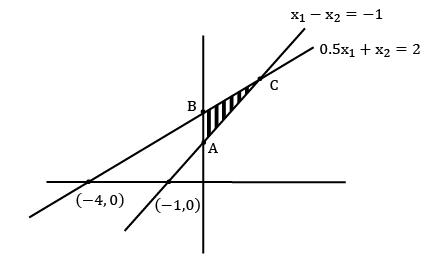zA = (0, 1) = 2

zB = (0, 2) = 4∗

zC = (2, 3) = 4∗

QUESTION: 2

### Solve the following LPP and identify the type of solutionMax z = 3x1 + 4x2s/t: x1 − x2 ≥ 02.5x1 − x2 ≤ −3x1 , x2 ≥ 0

Solution: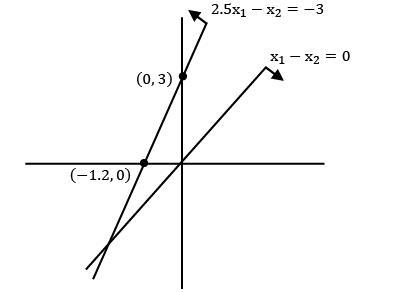No feasible region

*Answer can only contain numeric values
QUESTION: 3

### A company produces cups and trays by deep drawing and forming operations. Following data is given on capacity availability and economics of each product.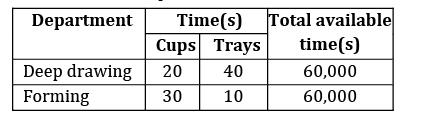The profit on each cup and tray is given as Rs.40 and Rs.80 respectively. Total daily fixed cost is Rs.90, 000. The maximum net profit per day at the optimum production level is ____________ Rs. [Consider the effect of fixed cost also]Option~(A) 30,000(B) 30,000

Solution:

Let no. of cups = x

No. of trays = y

Fixed cost = 90,000

Max z = 40x + 80y − 90000

For deep drawing machine 20x + 40y ≤ 60,000

For forming machine 30x + 10y ≤ 60,000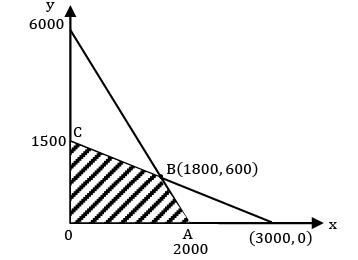z(0,0) = 0

z(0,1500) = 30,000

z(1800,600) = 30, 000

z(2000,0) = −10,000

QUESTION: 4

Consider the following LPP

Max z = 2x1 + 10x2

s/t: 3x1 + 2x2 ≤ 4

5x1 + 3x2 ≤ 6

x1 + x2 ≤ 10

The solution for the above LPP is

Solution:

Given,

Max z = 2x1 + 10x2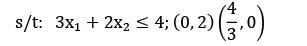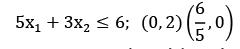x1 + x2 , ≤ 10; (0, 10)(10, 0)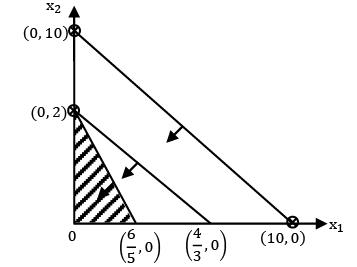z(0,0) = 0

z(0,2) = 20∗

z(6/5,0) = 2.4

i) One of the decision variable is zero

ii) There is a redundant constraint present but fully consumed. 3x1 + 2x2 + s1 = 4; s1 = 0

QUESTION: 5

Consider the following LPP

Minimize z = 5x1 + 7x2 + x3

s/t: 2x1 − x2 + 4x3 ≤ 10

x1 , x2 , x3 ≥ 0

The solution for the above LPP is

Solution:

Standard form:

z − 5x1 − 7x2 − x3 = 0

2x1 − x2 + 4x3 + s1 = 10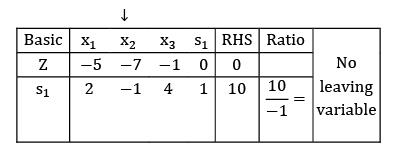QUESTION: 6

Consider the following primal LPP. Find dual of the given primal.

Max: z = 4x1 + 2x2 + 6x3

s/t: 2x1 + 3x2 + 2x3 ≥ 6

3x1 + 4x2 ≤ 8

6x1 − 4x2 + x3 ≤ 10

s/ t: 2y1 + 3y2 + 6y3 ≥ 4

3y1 + 4y2 − 4y3 ≥ 2

2y1 + y3 ≥ 6

y1 , y2, y3 ≥ 0

s/t: − 2y1 + 3y2 + 6y3 ≥ 4

−3y1 + 4y2 − 4y3 ≥ 2

−2y1 + y3 ≥ 6

y1 , y2, y3 ≥ 0

2y1 + 3y2 + 6y3 ≤ 4

3y1 + 4y2 − 4y3 ≤ 2

2y1 + y3 ≤ 6

y1 , y2, y3 ≥ 0

s/t: − 2y1 + 3y2 + 6y3 ≤ 4

−3y1 + 4y2 − 4y3 ≤ 2

−2y1 + y3 ≤ 6

y1 , y2, y3 ≥ 0

Solution:

zmax = 4x1 + 2x2 + 6x3

s/t: 2x1 + 3x2 + 2x3 ≥ 6

−2x1 − 3x2 − 2x3 ≤ −6

3x1 + 4x2 ≤ 8

6x1 − 4x2 + x3 ≤ 10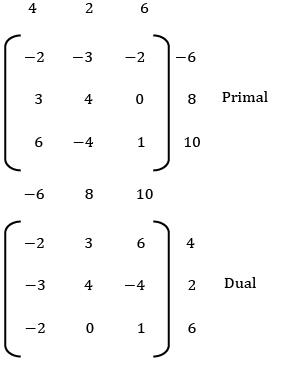Dual:

Min: w = −6y1 + 8y2 + 10y3

s/t: − 2y1 + 3y2 + 6y3 ≥ 4

−3y1 + 4y2 − 4y3 ≥ 2

−2y1 + y3 ≥ 6

y1 , y2 , y3 ≥ 0

QUESTION: 7

Find the initial basic feasible solution using VAM method.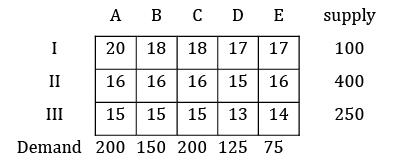Solution: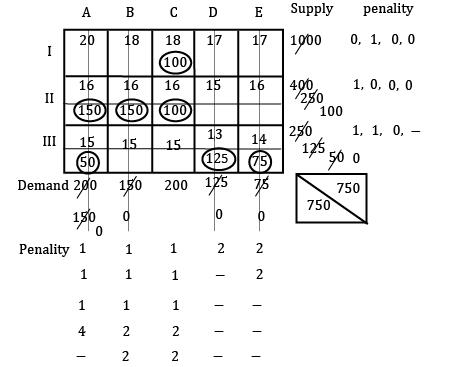TC = 18 × 100 + 150 × 16 + 150 × 16 + 16× 100 + 15 × 50 + 125 × 13 + 14 × 75

= 11, 625 Rs.

QUESTION: 8

A product is manufactured using two raw materials A and B. A is taken on x-axis and B is taken on Y-axis and the LPP is as follows.

Zmin = 2A + 3B

S⁄t ∶ 0.6 A + 0.3 B ≥ 45

0.1 A + 0.5B ≥ 25

A, B ≥ 0

Which of the following statement is correct?

Solution:
*Answer can only contain numeric values
QUESTION: 9

Four workers are required to do four jobs. Time to complete every job is given in the table below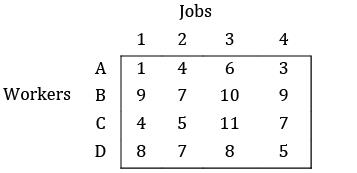Find the minimum cost.

Solution:

Row Reduction: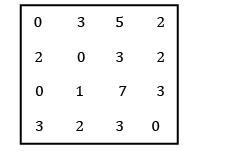Column Reduction: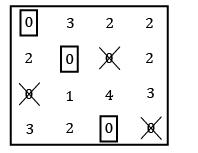Using straight line method: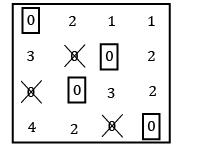TC = 1 + 10 + 5 + 5 = 21

*Answer can only contain numeric values
QUESTION: 10

Find optimal assignment cost.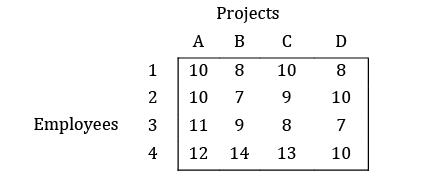Solution:

Row Reduction: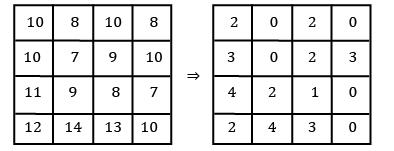Column Reduction: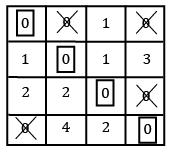It has multiple solutions TC = 10 + 7 + 8 + 10 = 35

*Answer can only contain numeric values
QUESTION: 11

Consider the following profit matrix. Find the max profit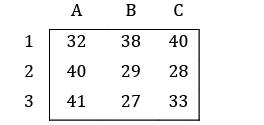Solution:

Row Reduction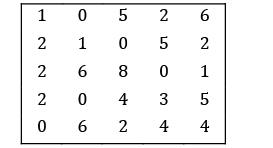Column Reduction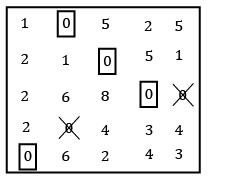Use straight line method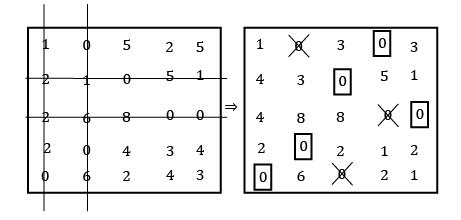Total cost = 4 + 3 + 2 + 2 + 2 = 13

*Answer can only contain numeric values
QUESTION: 12

Consider the problem of assigning four sales persons to four different sales regions as given below. Find the maximum total sales?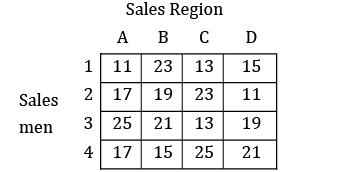All the values are given in thousands.

Solution:

The given problem is maximization problem. Hungarian algorithm is applicable to only minimization problem so, convert the problem into minimization by subtracting all the elements from max element.

Max element is ‘25’

Loss matrix: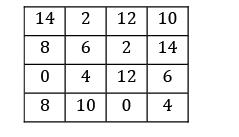i) Row Reduction: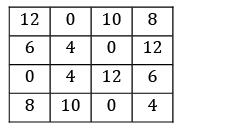ii) Column Reduction: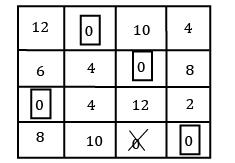Total sales = 23 + 23 + 25 + 21 = 92

*Answer can only contain numeric values
QUESTION: 13

Consider the following problem A cost matrix is given, find the minimum total cost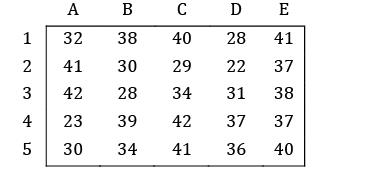Solution:

i) Row Reduction: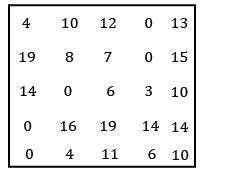ii) Column Reduction: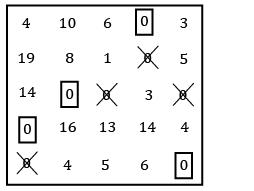No zero available for allocation ⇒ use straight line method.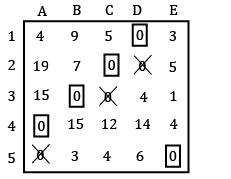TC = 28 + 29 + 28 + 23 + 40 = 148

*Answer can only contain numeric values
QUESTION: 14

Three workers (P, Q, R) are assigned to 3 machines (M1 , M2 , M3 ) while operator Q cannot be assigned machine M2 . Further, each operator is assigned to one machine and one machine requires only one operator. The operating cost is given for these operators in table below (in Rs). The minimum cost of assignment is ___________ (Rs).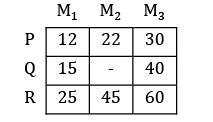Solution: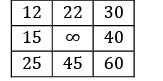Row Reduction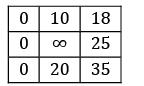Column Reduction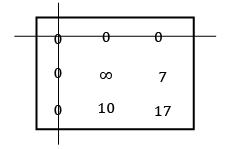Use straight line method: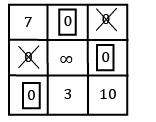Total minimum cost = 22 + 40 + 25 = 87